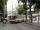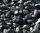# Reconstruction of the corridor

Calculate how many minutes will be reduced to travel 187 km long railway corridor, where the maximum speed increases from 120 km/h to 160 km/h.

Calculate how many minutes will shorten travel time, if we consider that the train must stop at 6 stations, each station with the equally slow 0.8 m/s2 and from station accelerates 0.5 m/s2?

What is the average speed before and after reconstruction, if train stay for 2 minutes in every station?

Consider the intermediate stops are so long that the train will always reach speed limit.

Result

t1 =  23.375 min
t2 =  20.944 min
v1 =  0 km/h
v2 =  0 km/h

#### Solution:

$t_{ 1 } = 60 \cdot \ (187/120-187/160) = \dfrac{ 187 }{ 8 } = 23.375 = 23.375 \ \text { min }$
$a_{ 1 } = 0.8 \cdot \ 3600 \cdot \ 3600/1000 = 10368 \ \\ a_{ 2 } = 0.5 \cdot \ 3600 \cdot \ 3600/1000 = 6480 \ \\ t_{ 11 } = 120/a_{ 1 } = 120/10368 = \dfrac{ 5 }{ 432 } \doteq 0.0116 \ \\ t_{ 21 } = 160/a_{ 1 } = 160/10368 = \dfrac{ 5 }{ 324 } \doteq 0.0154 \ \\ t_{ 12 } = 120/a_{ 2 } = 120/6480 = \dfrac{ 1 }{ 54 } \doteq 0.0185 \ \\ t_{ 22 } = 160/a_{ 2 } = 160/6480 = \dfrac{ 2 }{ 81 } \doteq 0.0247 \ \\ \ \\ s_{ 11 } = a_{ 1 } \cdot \ t_{ 11 }^{ 2 }/2 = 10368 \cdot \ 0.0116^{ 2 }/2 = \dfrac{ 25 }{ 36 } \doteq 0.6944 \ \\ s_{ 21 } = a_{ 1 } \cdot \ t_{ 21 }^{ 2 }/2 = 10368 \cdot \ 0.0154^{ 2 }/2 = \dfrac{ 10 }{ 9 } \doteq 1.1111 \ \\ \ \\ s_{ 21 } = a_{ 2 } \cdot \ t_{ 12 }^{ 2 }/2 = 6480 \cdot \ 0.0185^{ 2 }/2 = \dfrac{ 10 }{ 9 } \doteq 1.1111 \ \\ s_{ 22 } = a_{ 2 } \cdot \ t_{ 22 }^{ 2 }/2 = 6480 \cdot \ 0.0247^{ 2 }/2 = \dfrac{ 160 }{ 81 } \doteq 1.9753 \ \\ \ \\ T_{ 1 } = (7 \cdot \ (t_{ 11 }+t_{ 12 })+ (187-7 \cdot \ (s_{ 11 }+s_{ 21 }))/120) = (7 \cdot \ (0.0116+0.0185)+ (187-7 \cdot \ (0.6944+1.1111))/120) \doteq 1.6637 \ \\ T_{ 2 } = (7 \cdot \ (t_{ 21 }+t_{ 22 })+ (187-7 \cdot \ (s_{ 21 }+s_{ 22 }))/160) = (7 \cdot \ (0.0154+0.0247)+ (187-7 \cdot \ (1.1111+1.9753))/160) = \dfrac{ 631 }{ 480 } \doteq 1.3146 \ \\ t_{ 2 } = 60 \cdot \ (T_{ 1 }-T_{ 2 }) = 60 \cdot \ (1.6637-1.3146) = \dfrac{ 377 }{ 18 } \doteq 20.9444 = 20.944 \ \text { min }$
$v_{ 1 } = 187/(T_{ 1 }+6/30) = 187/(1.6637+6/30) \doteq 100.3403 = 0 \ \text { km/h }$
$v_{ 2 } = 187/(T_{ 2 }+6/30) = 187/(1.3146+6/30) \doteq 123.4663 = 0 \ \text { km/h }$

Our examples were largely sent or created by pupils and students themselves. Therefore, we would be pleased if you could send us any errors you found, spelling mistakes, or rephasing the example. Thank you!

Leave us a comment of this math problem and its solution (i.e. if it is still somewhat unclear...):Be the first to comment!#### Following knowledge from mathematics are needed to solve this word math problem:

Do you have a linear equation or system of equations and looking for its solution? Or do you have quadratic equation? Do you want to convert length units?

## Next similar math problems:

1. TrolleybusTrolleybus line No. 206 measured 24 km. If the trolleybus goes faster by 5 km/h, the way there and back would is shorter by 33 minutes. Calculate the trolleybus speed and how much time it takes a return trip.
2. ŽSRCalculate fixed annual personnel costs of operating monorail line 118 km long if every 5 km is station, which serve three people - one dispatcher and two switchman in 4-shift operation. Consider the average salary of the employee 885 €.
3. Climb in percentageThe height difference between points A and B is 475 m. Calculate the percentage of route climbing if the horizontal distance places A, B is 7.4 km.
4. Cu thiefThe thief stole 122 meters copper wire with cross-section area of 95 mm2. Calculate how much money gets in the scrap redemption, if redeemed copper for 5.5 eur/kg? The density of copper is 8.96 t/m3.
5. Earth parallelEarth's radius is 6370 km long. Calculate the length parallel of latitude 50°.
6. Motion problemFrom Levíc to Košíc go car at speed 81 km/h. From Košíc to Levíc go another car at speed 69 km/h. How many minutes before the meeting will be cars 27 km away?
7. PoolThe swimming pool is 10 m wide and 8 m long and 153 cm deep. How many hectoliters of water is in it, if the water is 30 cm below its upper edge?
8. TanksFire tank has cuboid shape with a rectangular floor measuring 13.7 m × 9.8 m. Water depth is 2.4 m. Water was pumped from the tank into barrels with a capacity of 2.7 hl. How many barrels were used, if the water level in the tank fallen 5 cm? Wr
9. The potThe pot is in 1/3 filled with water. Bottom of the pot has an area of ​​329 cm2. How many centimeters rises water level in the pot after add 1.2 liters of water?
10. CokeFrom one ton of coal is produced 772 kg of coke for iron production. How many wagons of coal by 13 tonnes per day is needed for the blast furnace, which has a daily consumption of 1020 tons of coke?
11. Bicycle wheelA bicycle wheel has a diameter of 60 cm. Approximately how many times does wheel rotate at 2.5 km long trip?
12. MotionIf you go at speed 3.7 km/h, you come to the station 42 minutes after leaving the train. If you go by bike to the station at speed 27 km/h, you come to the station 56 minutes before its departure. How far is the train station?
13. Motion2Cyclist started out of town at 19 km/h. After 0.7 hours car started behind him in the same direction and caught up with him for 23 minutes. How fast and how long went car from the city to caught cyclist?
14. TimeWrite time in minutes rounded to one decimal place: 5 h 28 m 26 s.
15. RectangleThe rectangle is 21 cm long and 38 cm wide. Determine the radius of the circle circumscribing rectangle.
16. GutterHow much metal is needed for production 60 pieces of gutter pipes with the diameter 17 cm and length of 6 m? The plate bends add 6% of the material.
17. Density - simple exampleMaterial of density of 532 kg/m3 occupies a container volume of 79 cm3. What is its mass?